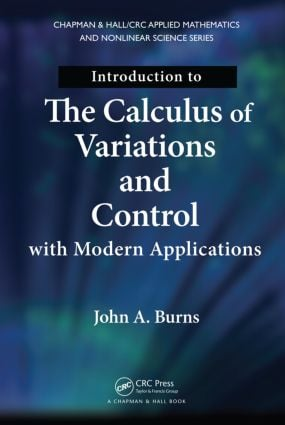# Introduction to the Calculus of Variations and Control with Modern Applications

## 1st Edition

Chapman and Hall/CRC

562 pages | 59 B/W Illus.

##### Purchasing Options:\$ = USD
Hardback: 9781466571396
pub: 2013-08-28
SAVE ~\$27.00
\$135.00
\$108.00
x
eBook (VitalSource) : 9780429190216
pub: 2013-08-28
from \$28.98

FREE Standard Shipping!

### Description

Introduction to the Calculus of Variations and Control with Modern Applications provides the fundamental background required to develop rigorous necessary conditions that are the starting points for theoretical and numerical approaches to modern variational calculus and control problems. The book also presents some classical sufficient conditions and discusses the importance of distinguishing between the necessary and sufficient conditions.

In the first part of the text, the author develops the calculus of variations and provides complete proofs of the main results. He explains how the ideas behind the proofs are essential to the development of modern optimization and control theory. Focusing on optimal control problems, the second part shows how optimal control is a natural extension of the classical calculus of variations to more complex problems.

By emphasizing the basic ideas and their mathematical development, this book gives you the foundation to use these mathematical tools to then tackle new problems. The text moves from simple to more complex problems, allowing you to see how the fundamental theory can be modified to address more difficult and advanced challenges. This approach helps you understand how to deal with future problems and applications in a realistic work environment.

### Table of Contents

Calculus of Variations

Historical Notes on the Calculus of Variations

Some Typical Problems

Some Important Dates and People

Introduction and Preliminaries

Motivating Problems

Mathematical Background

Function Spaces

Mathematical Formulation of Problems

The Simplest Problem in the Calculus of Variations

The Mathematical Formulation of the SPCV

The Fundamental Lemma of the Calculus of Variations

The First Necessary Condition for a Global Minimizer

Implications and Applications of the FLCV

Necessary Conditions for Local Minima

Weak and Strong Local Minimizers

The Euler Necessary Condition - (I)

The Legendre Necessary Condition - (III)

Jacobi Necessary Condition - (IV)

Weierstrass Necessary Condition - (II)

Applying the Four Necessary Conditions

Sufficient Conditions for the Simplest Problem

A Field of Extremals

The Hilbert Integral

Fundamental Sufficient Results

Summary for the Simplest Problem

Extensions and Generalizations

Properties of the First Variation

The Free Endpoint Problem

The Simplest Point to Curve Problem

Vector Formulations and Higher Order Problems

Problems with Constraints: Isoperimetric Problem

Problems with Constraints: Finite Constraints

An Introduction to Abstract Optimization Problems

Applications

Solution of the Brachistochrone Problem

Classical Mechanics and Hamilton's Principle

A Finite Element Method for the Heat Equation

Optimal Control

Optimal Control Problems

An Introduction to Optimal Control Problems

The Rocket Sled Problem

Problems in the Calculus of Variations

Time Optimal Control

Simplest Problem in Optimal Control

SPOC: Problem Formulation

The Fundamental Maximum Principle

Application of the Maximum Principle to Some Simple Problems

Extensions of the Fundamental Maximum Principle

A Fixed-Time Optimal Control Problem

Application to Problems in the Calculus of Variations

Application to the Farmer’s Allocation Problem

Application to a Forced Oscillator Control Problem

Application to the Linear Quadratic Control Problem

The Maximum Principle for a Problem of Bolza

The Maximum Principle for Nonautonomous Systems

Application to the Nonautonomous LQ Control Problem

Linear Control Systems

Introduction to Linear Control Systems

Linear Control Systems Arising from Nonlinear Problems

Linear Quadratic Optimal Control

The Riccati Differential Equation for a Problem of Bolza

Estimation and Observers

The Time Invariant Infinite Interval Problem

The Time Invariant Min-Max Controller

Problems appear at the end of each chapter.

### About the Author

John Burns is the Hatcher Professor of Mathematics, Interdisciplinary Center for Applied Mathematics at Virginia Polytechnic Institute and State University. He is a fellow of the IEEE and SIAM. His research interests include distributed parameter control; approximation, control, identification, and optimization of functional and partial differential equations; aero-elastic control systems; fluid/structural control systems; smart materials; optimal design; and sensitivity analysis.

### Subject Categories

##### BISAC Subject Codes/Headings:
MAT003000
MATHEMATICS / Applied
MAT007000
MATHEMATICS / Differential Equations
TEC007000
TECHNOLOGY & ENGINEERING / Electrical